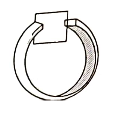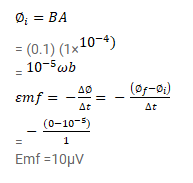# The given figure shows a conducting square loop placed parallel

Question:

The given figure shows a conducting square loop placed parallel to the pole-faces of a ring magnet. The pole-faces have an area of $1 \mathrm{~cm}^{2}$ each and the field between the poles is $0.10 \mathrm{~T}$. The wires making the loop are all outside the magnetic field. If the magnet is removed in $1.0 \mathrm{~s}$. What is the average emf induced in the loop?Solution: